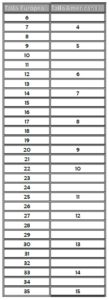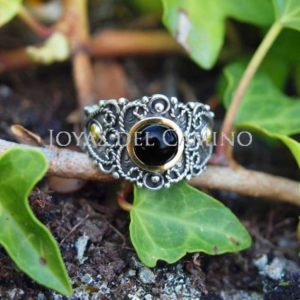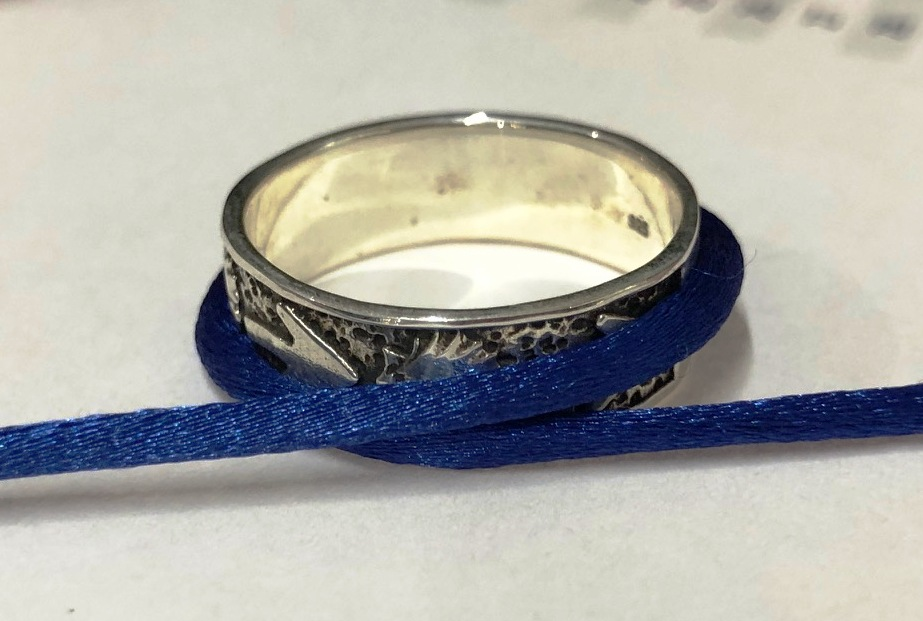To calculate the size of your ring there are two ways:

• Find a ring that you wear regularly and measure the inside diameter of the ring.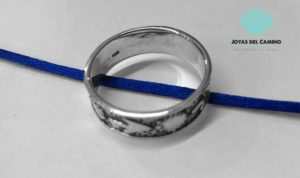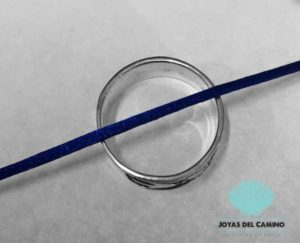With this measurement we consult the following table where we will find the size that corresponds to it.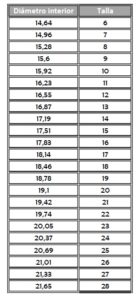• If we don’t have a ring, we can measure the circumference of the finger. The easiest way is to do it with a cord that we will later measure to find the exact figure. We then consult the table below to see the equivalence.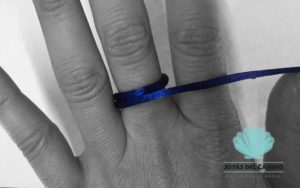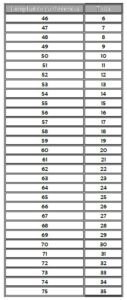The equivalences between ring measurements with European Size and American Size are the following: